## Welcome to K C Yan's Singapore Math blog!

Wanting to be updated on Singapore Math news or new Singapore Math? You have come at the right place! Please leave your comments before leaving. A googol thanks.

## Sunday, March 31, 2013

### The Mathematics of the Cross

Amazing love! How can it be
that thou, my God, shouldst die for me?Flag of Red Cross
The cross isn't just a sacred icon or symbol for religious math educators, but it's also a geometrical shape that has borne much fruit in producing many logic and mathematics puzzles. Few recreational mathematicians, professional and amateur, wouldn't want to be challenged by these fertile dissection puzzles.Flag of Switzerland
Let's resurrect some of these ancient cross puzzles, which have given so much intellectual satisfaction to those who have decided to take up their cross along their mathematical itinerary. Below are ten of these Christmaths puzzles, which would appeal to above-average elementary and middle-school math students and their teachers.

1. Squares in a Cross

A solid Greek cross can be formed by putting together five cubes, or from a number of squares. How many squares are there?

2. Tessellating and Dissecting Crosses

(a) Show how Greek crosses can form a tessellation.

(b) How can an infinite number of dissections from a cross tessellation produce a square?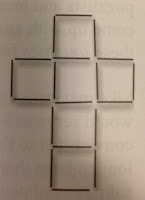3. A matchstick Puzzle

The cross on the left is made up of 19 matches. Move 7 of them to make a pattern consisting of four squares.

4. The Area of a Cross

A cross is made up of five congruent squares. If XY = 10 cm, what is the area of the cross?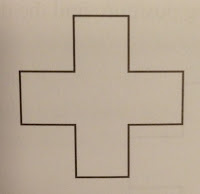5. Cross into Rectangle

Using only two straight cuts, divide the cross on the right into three pieces and reassemble them to form a rectangle twice as long as it is wide.

6. Five-piece Square into Cross

Cut a square into five pieces and rearrange them to form a Greek cross, as shown below.

7. Four-piece Square into Cross

Cut a square into four pieces and rearrange them to form a Greek cross, as shown below.8. Cross into Hollow Square

The Greek cross on the left has a square-shaped hole in the center.
(a) Rearrange the pieces to make a square that has a hollow cross inside.
(b) Rearrange the pieces so that the resulting figure is a square that is rather smaller than the previous "hollow" one.

9. The Cross and the Crescent

Reassemble the seven pieces of the crescent to make the Greek cross.

10. The Rolling Disc

In the figure below, each side of the cross is 10 cm long. A small circular disc of radius 1 cm is placed at one corner. If the disc rolls along the sides of the figure and returns to the starting position, find the distance traveled by the center of the disc.

1. 22 squares.
4. 100 cm².
5.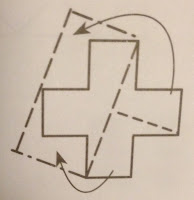7.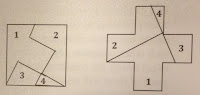8.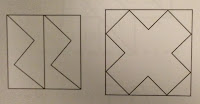10. (104 + 2π) cm

Reference
Yan, K. C. (2011). CHRISTmaths: A creative problem solving math book. Singapore: MathPlus Publishing.

© Yan Kow Cheong, March 31, 2013.

Code: WR3ZTVKVUW9Z